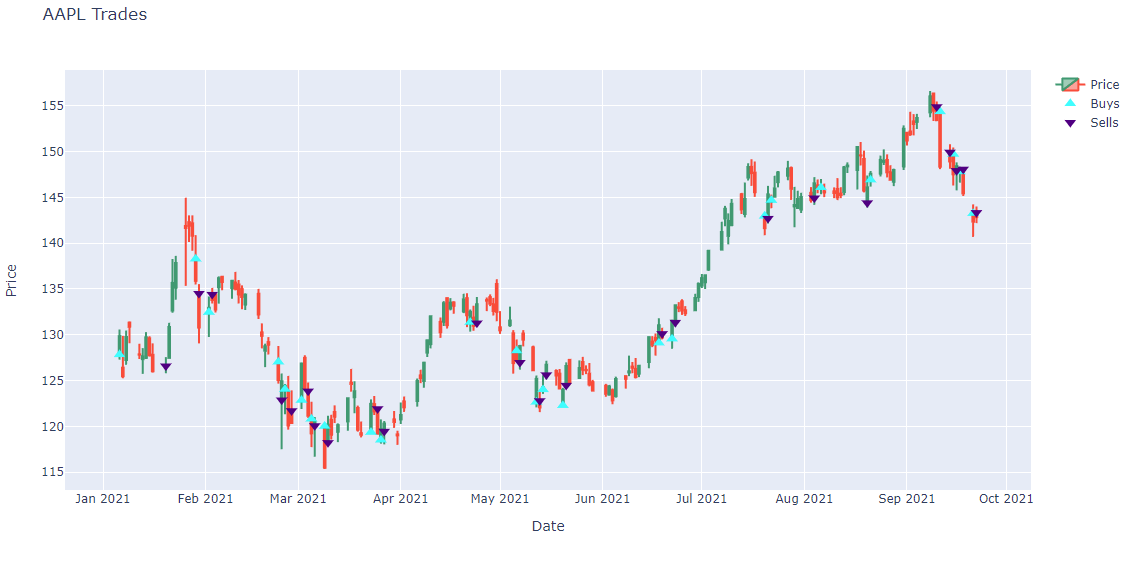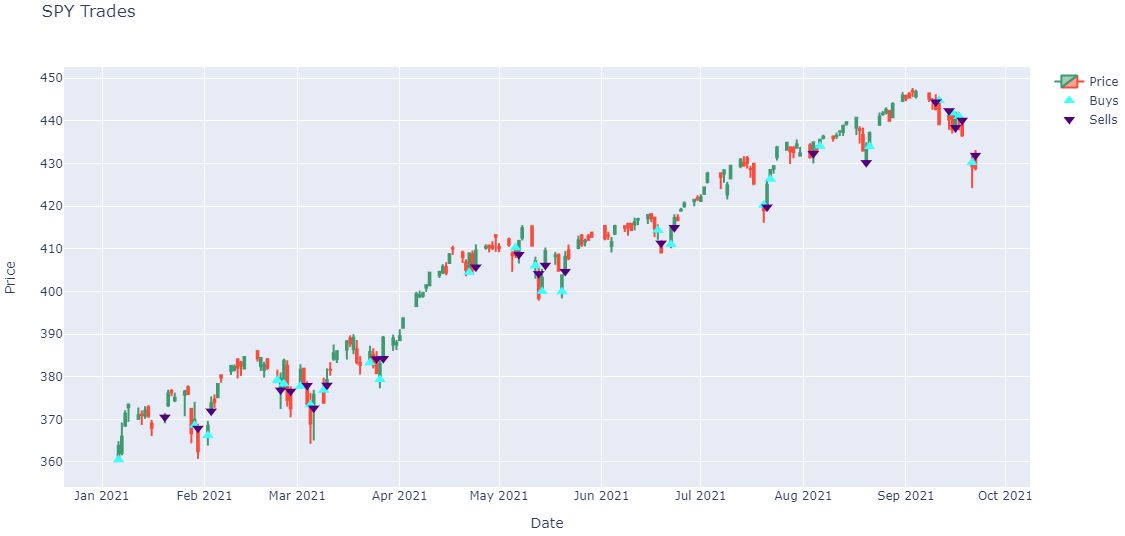# Live Algorithms

### Introduction

This page explains how to read live trading orders into the Research Environment and plot the trades.

### Prerequisites

Working knowledge of Python and pandas DataFrames. If you are not familiar with pandas, refer to the pandas documentation.

### Get Orders

To get the orders of a backtest, call the ReadLiveOrders method.

orders = api.ReadLiveOrders(project_id)

By default, the orders with an ID between 0 and 100. To get orders with an ID greater than 100, pass start and end arguments to the ReadLiveOrders method. Note that end - start must be less than 100.

orders = api.ReadLiveOrders(project_id, 100, 150)

### Order Properties

Order objects have the following properties:

### Plot Fills

You need to get your live trading orders to plot your order fills.

Follow these steps to plot the daily buy and sell fill prices for the securities in your algorithm:

1. Organize the trade times and prices for each security into a dictionary.
class OrderData:
def __init__(self):
self.sell_fill_times = []
self.sell_fill_prices = []

order_data_by_symbol = {}
for order in orders:
if order.Symbol not in order_data_by_symbol:
order_data_by_symbol[order.Symbol] = OrderData()
order_data = order_data_by_symbol[order.Symbol]
is_buy = order.Quantity > 0
(order_data.buy_fill_prices if is_buy else order_data.sell_fill_prices).append(order.Price)
2. Get the price history of each security you traded.
qb = QuantBook()
start_date = datetime.max.date()
end_date = datetime.min.date()
for symbol, order_data in order_data_by_symbol.items():
start_date = min(start_date, min(order_data.buy_fill_times), min(order_data.sell_fill_times))
end_date = max(end_date, max(order_data.buy_fill_times), max(order_data.sell_fill_times))
start_date -= timedelta(days=1)
all_history = qb.History(list(order_data_by_symbol.keys()), start_date, end_date, Resolution.Daily)
3. Create a candlestick plot for each security and annotate each plot with buy and sell markers.
import plotly.express as px
import plotly.graph_objects as go

for symbol, order_data in order_data_by_symbol.items():
history = all_history.loc[symbol]

# Plot security price candlesticks
candlestick = go.Candlestick(x=history.index,
open=history['open'],
high=history['high'],
low=history['low'],
close=history['close'],
name='Price')
layout = go.Layout(title=go.layout.Title(text=f'{symbol.Value} Trades'),
xaxis_title='Date',
yaxis_title='Price',
xaxis_rangeslider_visible=False,
height=600)
fig = go.Figure(data=[candlestick], layout=layout)

marker=go.scatter.Marker(color='aqua', symbol='triangle-up', size=10),
mode='markers',
))

# Plot sells
x=order_data.sell_fill_times,
y=order_data.sell_fill_prices,
marker=go.scatter.Marker(color='indigo', symbol='triangle-down', size=10),
mode='markers',
name='Sells',
))

fig.show()Note: The preceding plots only show the last fill of each trade. If your trade has partial fills, the plots only display the last fill.

You can also see our Videos. You can also get in touch with us via Discord.